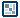## Question of the WeekCreating Equilibrium Points
by Christian Eilsøe

Introduction
In this tutorial, we'll take a gander at what simple mathematics can accomplish when used right. =0) With simple math, we can do all sorts of stuff, i.e. EQ points. IF you paid attention in math classes in grade school, this won't be new to you.

Below is an example of how EQ points work. Just drag the red balls around, and notice how the square relates to the balls.

[ Example of EQ points in flash: drag the circles around]

Let's get on it then:

1. Create a circle on the main timeline, make it a movie clip, and name it "ball1".

2. Add the following code to ball1 in the actions panel:

on(press){
startDrag(this);
}
on(release){
stopDrag();
}

3. When done, select ball1, and press ctrl+D three times, to duplicate the clip. Then we should have 4 red balls on the stage =0)

4. Rename the instances, so we have ball1, ball2, ball3 and ball4.
1. Nextly, create a small square in the middle of our stage. You don't have to name this one =0)
Call it the "Evil Lawnmover from hell" for all I care *lol*.

2. We SHOULD now have something similar to what I have down below:3. Allrighty, time to code the square.
IF you'd listened carefully in grade school, you'll know, that finding the "middle" value between two other values, is quite simple. Let's try it:

value1= 450, value2=325

EQ value = (450+325)/2 = 387.5

See, the only thing I had to do, was add the values together, and divide by the number of total values I had! =0)   Easy huh?

4. Ok now, let's make the square move!
As mentioned above, we have to add all values and divide by the number of values we have, so, think!
We have 4 balls, and we want the square to position itself at the "middle" point of all 4 balls. So actually, all we have to do, is add all the x values and thereafter, all the y values =0)

Add the following code to the square:

onClipEvent(enterframe){
xpos=(_root.ball1._x+_root.ball2._x+_root.ball3._x+_root.ball4._x)/4;

ypos=(_root.ball1._y+_root.ball2._y+_root.ball3._y+_root.ball4._y)/4;
_x=xpos;
_y=ypos;
}

So there we have it =0)
Test your movie and drag around the circles!

-Christian Eilsøe a.k.a. eilsoe
-e-mail: [email protected]-
-Homepage: http://www.avalon-rev.dk